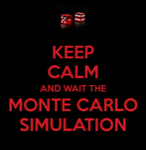Cococubed.com Monte Carlo Massive Stars

Home

Astronomy research
Software Infrastructure:
MESA
FLASH
STARLIB
MESA-Web
starkiller-astro
My instruments
White dwarf supernova:
Remnant metallicities
Colliding white dwarfs
Merging white dwarfs
Ignition conditions
Metallicity effects
Central density effects
Detonation density effects
Tracer particle burning
Subsonic burning fronts
Supersonic burning fronts
W7 profiles
Massive star supernova:
Rotating progenitors
3D evolution
26Al & 60Fe
44Ti, 60Co & 56Ni
Effects of 12C +12C
SN 1987A light curve
Constraints on Ni/Fe ratios
An r-process
Neutron Stars and Black Holes:
Black Hole Mass Gap
Compact object IMF
Stars:
Neutrino HR diagram
Pulsating white dwarfs
Pop III with JWST
Monte Carlo massive stars
Neutrinos from pre-SN
Pre-SN variations
Monte Carlo white dwarfs
SAGB stars
Classical novae
He shell convection
Presolar grains
He burn on neutron stars
BBFH at 40 years
Chemical Evolution:
Iron Pseudocarbynes
Hypatia catalog
Zone models H to Zn
Mixing ejecta
γ-rays within 100 Mpc
Thermodynamics & Networks
Stellar EOS
12C(α,γ)16O Rate
Proton-rich NSE
Reaction networks
Bayesian reaction rates
Verification Problems:
Validating an astro code
Su-Olson
Cog8
RMTV
Sedov
Noh
Software instruments
Presentations
Illustrations
Public Outreach
Education materials
2022 ASU Solar Systems Astronomy
2022 ASU Energy in Everyday Life

AAS Journals
2022 Earendel, A Highly Magnified Star
2022 TV Columbae, Micronova
2022 White Dwarfs and 12C(α,γ)16O
2022 MESA in Don't Look Up
2022 MESA Marketplace
2022 MESA Summer School
2022 MESA Classroom
2021 Bill Paxton, Tinsley Prize

Contact: F.X.Timmes
my one page vitae,
full vitae,
research statement, and
teaching statement.
The Impact of Nuclear Reaction Rate Uncertainties On The Evolution of Core-Collapse Supernova Progenitors (2018)

In this article we explore properties of core-collapse supernova progenitors with respect to the composite uncertainties in the thermonuclear reaction rates by coupling the reaction rate probability density functions provided by the STARLIB reaction rate library with MESA stellar models.

We evolve 1000 15 M$_{\odot}$ models from the pre main-sequence to core O-depletion at solar and subsolar metallicities for a total of 2000 Monte Carlo stellar models. For each stellar model, we independently and simultaneously sample 665 thermonuclear reaction rates and use them in a MESA in situ reaction network that follows 127 isotopes from $^{1}$H to $^{64}$Zn. With this framework we survey the core mass, burning lifetime, composition, and structural properties at five different evolutionary epochs. At each epoch we measure the probability distribution function of the variations of each property and calculate Spearman Rank-Order Correlation coefficients for each sampled reaction rate to identify which reaction rate has the largest impact on the variations on each property.

We find that uncertainties in $^{14}$N$(p,\gamma)^{15}$O, triple-$\alpha$, $^{12}$C$(\alpha,\gamma)^{16}$O, $^{12}$C($^{12}$C,p)$^{23}$Na, $^{12}$C($^{16}$O,p)$^{27}$Al, $^{16}$O($^{16}$O,n)$^{31}$S, $^{16}$O($^{16}$O,p)$^{31}$P, and $^{16}$O($^{16}$O,$\alpha$)$^{28}$Si reaction rates dominate the variations of the properties surveyed. We find that variations induced by uncertainties in nuclear reaction rates grow with each passing phase of evolution, and at core H-, He-depletion are of comparable magnitude to the variations induced by choices of mass resolution and network resolution. However, at core C-, Ne-, and O-depletion, the reaction rate uncertainties can dominate the variation causing uncertainty in various properties of the stellar model in the evolution towards iron core-collapse.